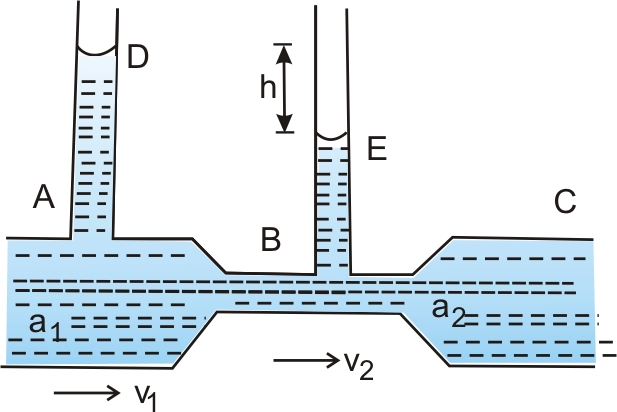# Which formula is used for measuring the rate of flow of liquid through pipes using the Venturimeter ??

Venturimeter is a device is used for measuring the rate of flow of liquid through pipes.

This formula is used to find the volume flow rate.For the above figure

a1 and a2 are an area of cross-section of tube A and B respectively

And v1 and v2 are the Velocities of the flow of liquid through A and B respectively.

Exams
Articles
Questions